Algebra 2 Worksheets

Quadratic Functions and Inequalities Worksheets

Here is a graphic preview for all of the Quadratic Functions and Inequalities Worksheets. You can select different variables to customize these Quadratic Functions and Inequalities Worksheets for your needs. The Quadratic Functions and Inequalities Worksheets are randomly created and will never repeat so you have an endless supply of quality Quadratic Functions and Inequalities Worksheets to use in the classroom or at home.

Our Quadratic Functions and Inequalities Worksheets are free to download, easy to use, and very flexible.

These Quadratic Functions and Inequalities Worksheets are a good resource for students in the 8th Grade through the 12th Grade.

Click here for a Detailed Description of all the Quadratic Functions and Inequalities Worksheets.

Quick Link for All Quadratic Functions and Inequalities Worksheets

Click the image to be taken to that Quadratic Functions and Inequalities Worksheets.

Properties of Parabolas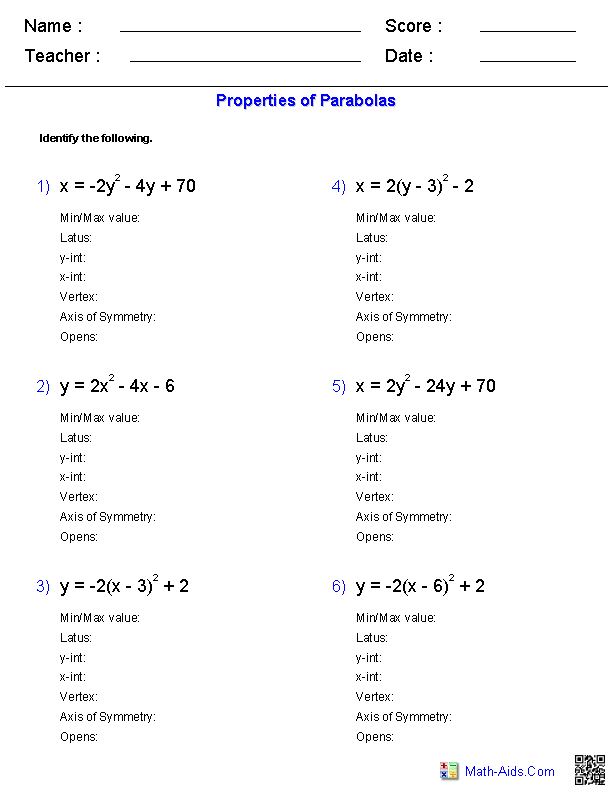Vertex Form of Parabolas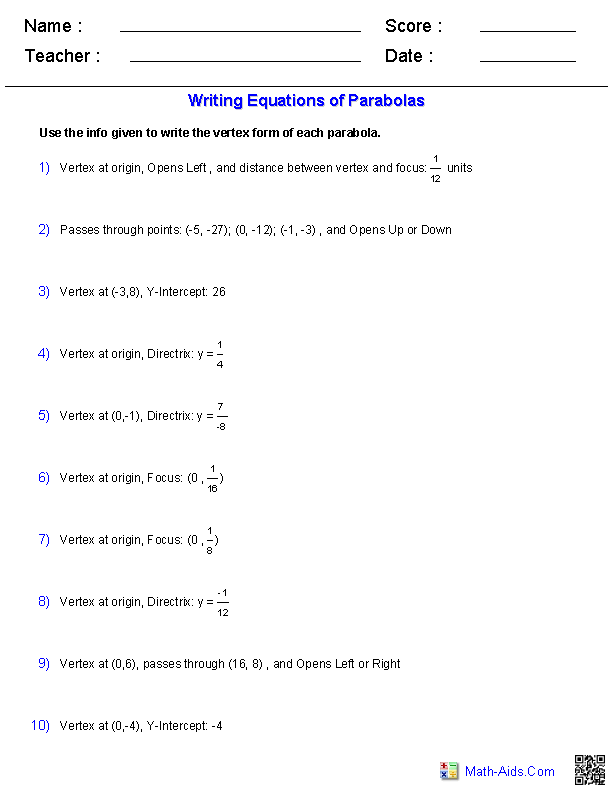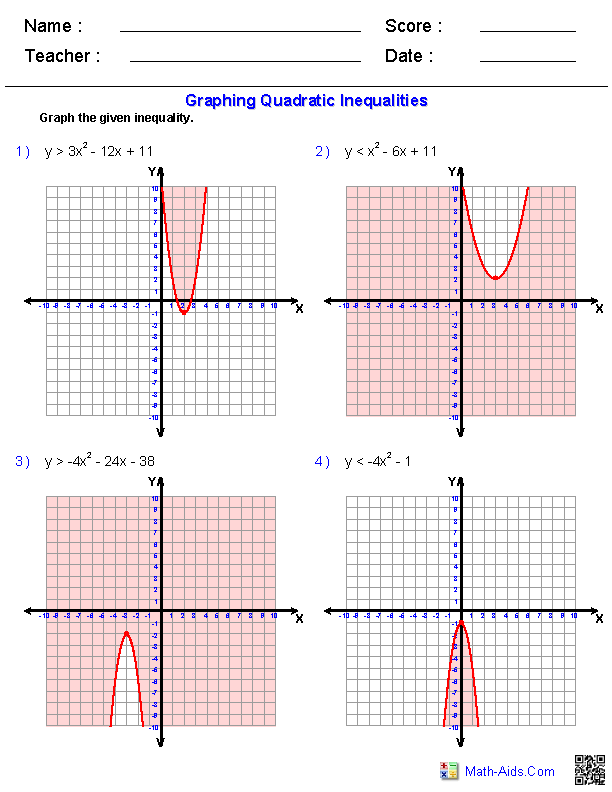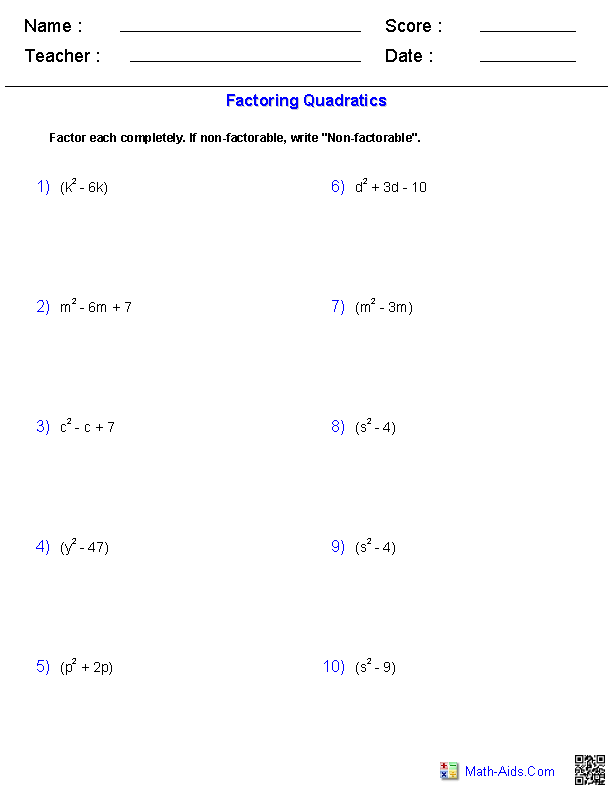Solving Quadratic Expressionswith Square Roots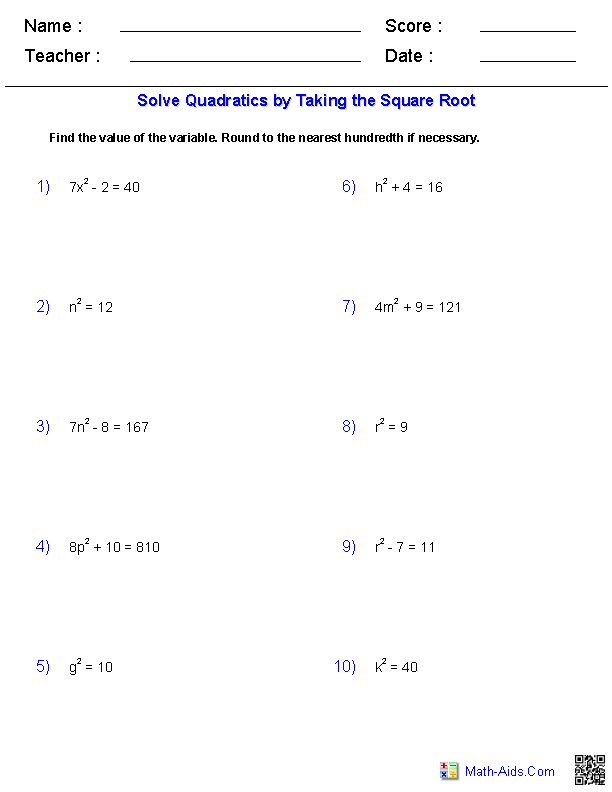Solving Quadratic Expressionsby FactoringCompletingthe Square Worksheets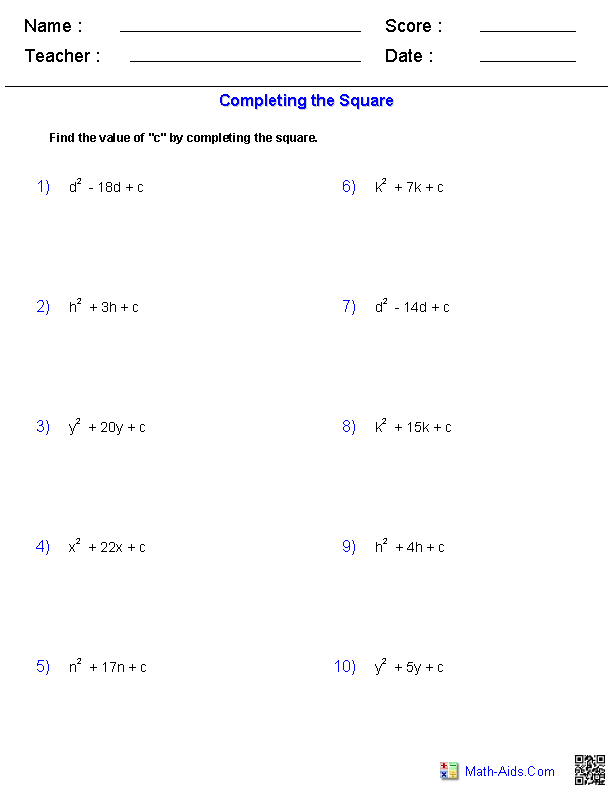Solving Equations byCompleting the Square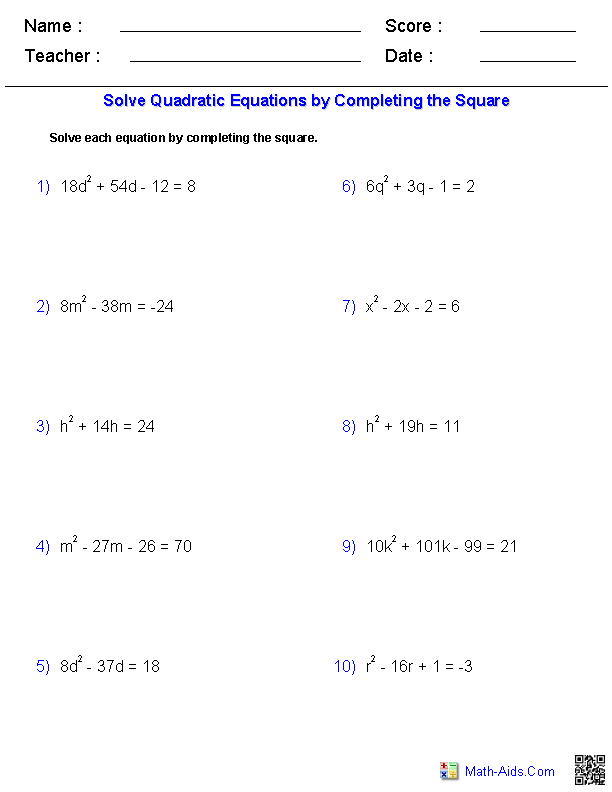Solving Equations withthe Quadratic Formula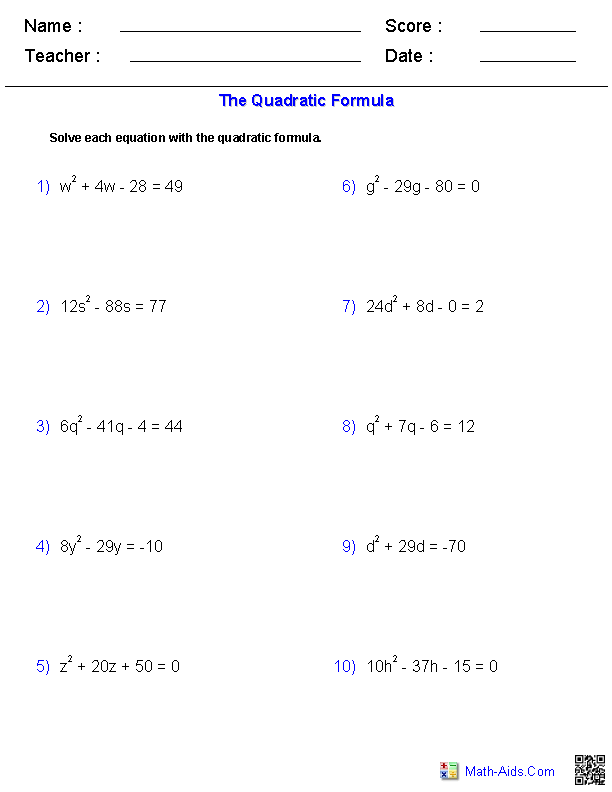Working withthe Discriminant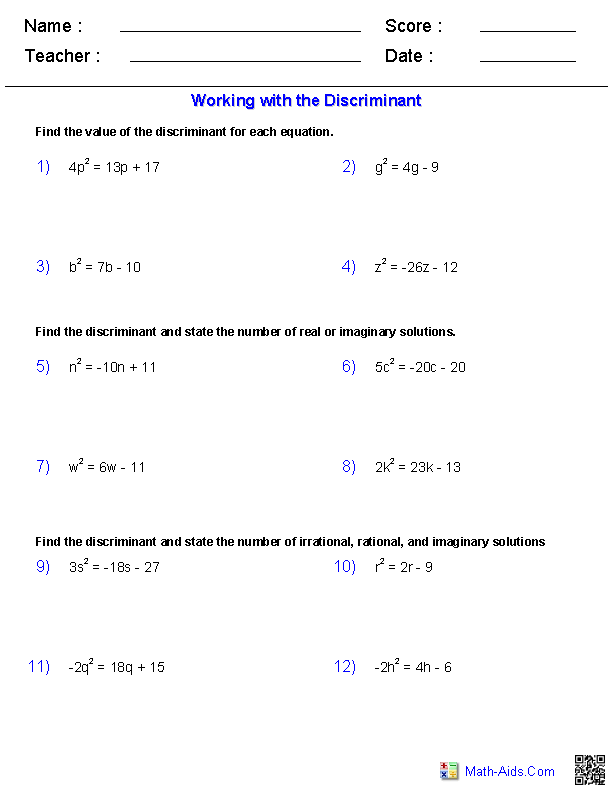Recommended Videos

Detailed Description for All Quadratic Functions and Inequalities Worksheets

Properties of Parabolas Worksheets
These Algebra 2 Quadratic Functions and Inequalities Worksheets will produce problems for properties of parabolas. You may select which properties to be identified from the equations. These Quadratic Functions and Inequalities Worksheets are a good resource for students in the 8th Grade through the 12th Grade.

Vertex Form of Parabolas Worksheets
These Algebra 2 Quadratic Functions and Inequalities Worksheets will produce problems for writing equations of parabolas. You may select the parabolas properties given to write the equation. These Quadratic Functions and Inequalities Worksheets are a good resource for students in the 8th Grade through the 12th Grade.

Graphing Quadratic Inequalities Worksheets
These Algebra 2 Quadratic Functions and Inequalities Worksheets produces problems for graphing quadratic inequalities. You can select the magnitude of the "a" term and the direction in which the parabola opens. These Quadratic Functions and Inequalities Worksheets are a good resource for students in the 8th Grade through the 12th Grade.

These Algebra 2 Quadratic Functions and Inequalities Worksheets will produce problems for factoring quadratic expressions. You may select which type of polynomials to factor, and whether you want some non-factorable expressions or not. These Quadratic Functions and Inequalities Worksheets are a good resource for students in the 8th Grade through the 12th Grade.

Solve by Taking the Square Root Worksheets
These Algebra 2 Quadratic Functions and Inequalities Worksheets produces problems for solving quadratic equations by taking the square root. You can select the difficulty of the problems and whether or not you always want a problem to have a solution. These Quadratic Functions and Inequalities Worksheets are a good resource for students in the 8th Grade through the 12th Grade.

Solve By Factoring Worksheets
These Algebra 2 Quadratic Functions and Inequalities Worksheets produces problems for solving quadratic equations by factoring. You can select the difficulty of the problems. These Quadratic Functions and Inequalities Worksheets are a good resource for students in the 8th Grade through the 12th Grade.

Complete the Square Worksheets
These Algebra 2 Quadratic Functions and Inequalities Worksheets will produce problems for completing the square. You may select the type of number for the b term. These Quadratic Functions and Inequalities Worksheets are a good resource for students in the 8th Grade through the 12th Grade.

Solve Quadratic Equations by Completing the Square Worksheets
These Algebra 2 Quadratic Functions and Inequalities Worksheets produces problems for solving quadratic equations by completing the square. You can select the difficulty of the problems and the types of roots. These Quadratic Functions and Inequalities Worksheets are a good resource for students in the 8th Grade through the 12th Grade.

Solve Quadratic Equations with the Quadratic Formula Worksheets
These Algebra 2 Quadratic Functions and Inequalities Worksheets produces problems for solving quadratic equations with the quadratic formula. You can select the difficulty of the problems and the types of roots. These Quadratic Functions and Inequalities Worksheets are a good resource for students in the 8th Grade through the 12th Grade.

Working with the Discriminant Worksheets
These Algebra 2 Quadratic Functions and Inequalities Worksheets will produce problems for working with the discriminant. You may select the types of quadratic equations and the value of the "a" term coefficient. These Quadratic Functions and Inequalities Worksheets are a good resource for students in the 8th Grade through the 12th Grade.